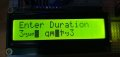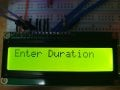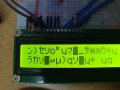# Matrix Keypad & 16*2 LCD interfacing with PIC

#### swsean

Joined Jul 8, 2019
22
Hi, guys. I am working on my final year project. I've been trying to interface the Matrix Keypad & 16*2 LCD along the with PIC. However, I wasn't able to get it to work. The LCD does not display the numbers entered.The microcontroller I used is PIC16F887. My current code is listed below. Please help me.

Code:
#pragma config CONFIG1 = 0x2CD4
#pragma config CONFIG2 = 0x0700

#include <stdio.h>
#include <stdlib.h>

//LCD module connections
#define LCD_RS       RD0
#define LCD_EN       RD1
#define LCD_D4       RD2
#define LCD_D5       RD3
#define LCD_D6       RD4
#define LCD_D7       RD5
#define LCD_RS_DIR   TRISD0
#define LCD_EN_DIR   TRISD1
#define LCD_D4_DIR   TRISD2
#define LCD_D5_DIR   TRISD3
#define LCD_D6_DIR   TRISD4
#define LCD_D7_DIR   TRISD5
//End LCD module connections

#define X_1    RB0
#define X_2    RB1
#define X_3    RB2
#define X_4    RB3
#define Y_1    RB4
#define Y_2    RB5
#define Y_3    RB6
#define Y_4    RB7

#include <xc.h>
#define _XTAL_FREQ 8000000
#include <stdint.h>        // include stdint header
#include "LCD_Lib.c"       // include LCD driver source file

// Function declarations
char switch_press_scan(void);

{
Keypad_PORT_Direction = 0xF0;    // Last 4 pins input, First 4 pins output

OPTION_REG &= 0x7F;
}

{
X_1 = 0; X_2 = 1; X_3 = 1; X_4 = 1;

if (Y_1 == 0) { __delay_ms(100); while (Y_1==0); return '1'; }
if (Y_2 == 0) { __delay_ms(100); while (Y_2==0); return '2'; }
if (Y_3 == 0) { __delay_ms(100); while (Y_3==0); return '3'; }
if (Y_4 == 0) { __delay_ms(100); while (Y_4==0); return 'A'; }

X_1 = 1; X_2 = 0; X_3 = 1; X_4 = 1;

if (Y_1 == 0) { __delay_ms(100); while (Y_1==0); return '4'; }
if (Y_2 == 0) { __delay_ms(100); while (Y_2==0); return '5'; }
if (Y_3 == 0) { __delay_ms(100); while (Y_3==0); return '6'; }
if (Y_4 == 0) { __delay_ms(100); while (Y_4==0); return 'B'; }

X_1 = 1; X_2 = 1; X_3 = 0; X_4 = 1;

if (Y_1 == 0) { __delay_ms(100); while (Y_1==0); return '7'; }
if (Y_2 == 0) { __delay_ms(100); while (Y_2==0); return '8'; }
if (Y_3 == 0) { __delay_ms(100); while (Y_3==0); return '9'; }
if (Y_4 == 0) { __delay_ms(100); while (Y_4==0); return 'C'; }

X_1 = 1; X_2 = 1; X_3 = 1; X_4 = 0;

if (Y_1 == 0) { __delay_ms(100); while (Y_1==0); return '*'; }
if (Y_2 == 0) { __delay_ms(100); while (Y_2==0); return '0'; }
if (Y_3 == 0) { __delay_ms(100); while (Y_3==0); return '#'; }
if (Y_4 == 0) { __delay_ms(100); while (Y_4==0); return 'D'; }

return 'n';
}

// Function name: GetKey
char switch_press_scan(void)                // Get key from user
{
char key = 'n';              // Assume no key pressed

while(key=='n')              // Wait until a key is pressed
key = keypad_scanner();   // Scan the keys again and again

return key;                  //when key pressed then return its value
}

void main(void)
{
LCD_Begin();
uint8_t t = 0;
static char Duration;
char Key = 'n';
LCD_Goto(1, 1);
LCD_Print("Enter Duration");
LCD_Goto(1, 2);
while(t<9){
Key = switch_press_scan();
Duration[t]=Key;
LCD_Goto(t+1, 2);
LCD_Print(Duration);
t++;
}
}
LCD library I used is:
Code:
#pragma warning disable 520

#include <stdint.h>

#define LCD_FIRST_ROW          0x80
#define LCD_SECOND_ROW         0xC0
#define LCD_THIRD_ROW          0x94
#define LCD_FOURTH_ROW         0xD4
#define LCD_CLEAR              0x01
#define LCD_RETURN_HOME        0x02
#define LCD_ENTRY_MODE_SET     0x04
#define LCD_CURSOR_OFF         0x0C
#define LCD_UNDERLINE_ON       0x0E
#define LCD_MOVE_CURSOR_LEFT   0x10
#define LCD_MOVE_CURSOR_RIGHT  0x14
#define LCD_TURN_ON            0x0C
#define LCD_TURN_OFF           0x08
#define LCD_SHIFT_LEFT         0x18
#define LCD_SHIFT_RIGHT        0x1E

#ifndef LCD_TYPE
#define LCD_TYPE 2           // 0=5x7, 1=5x10, 2=2 lines
#endif

__bit RS;

void LCD_Write_Nibble(uint8_t n);
void LCD_Cmd(uint8_t Command);
void LCD_Goto(uint8_t col, uint8_t row);
void LCD_PutC(char LCD_Char);
void LCD_Print(char* LCD_Str);
void LCD_Begin();

void LCD_Write_Nibble(uint8_t n)
{
LCD_RS = RS;
LCD_D4 = n & 0x01;
LCD_D5 = (n >> 1) & 0x01;
LCD_D6 = (n >> 2) & 0x01;
LCD_D7 = (n >> 3) & 0x01;

// send enable pulse
LCD_EN = 0;
__delay_us(1);
LCD_EN = 1;
__delay_us(1);
LCD_EN = 0;
__delay_us(100);
}

void LCD_Cmd(uint8_t Command)
{
RS = 0;
LCD_Write_Nibble(Command >> 4);
LCD_Write_Nibble(Command);
if((Command == LCD_CLEAR) || (Command == LCD_RETURN_HOME))
__delay_ms(2);
}

void LCD_Goto(uint8_t col, uint8_t row)
{
switch(row)
{
case 2:
LCD_Cmd(LCD_SECOND_ROW + col - 1);
break;
case 3:
LCD_Cmd(LCD_THIRD_ROW  + col - 1);
break;
case 4:
LCD_Cmd(LCD_FOURTH_ROW + col - 1);
break;
default:      // case 1:
LCD_Cmd(LCD_FIRST_ROW  + col - 1);
}

}

void LCD_PutC(char LCD_Char)
{
RS = 1;
LCD_Write_Nibble(LCD_Char >> 4);
LCD_Write_Nibble(LCD_Char );
}

void LCD_Print(char* LCD_Str)
{
uint8_t i = 0;
RS = 1;
while(LCD_Str[i] != '\0')
{
LCD_Write_Nibble(LCD_Str[i] >> 4);
LCD_Write_Nibble(LCD_Str[i++] );
}
}

void LCD_Begin()
{
RS = 0;

LCD_RS     = 0;
LCD_EN     = 0;
LCD_D4     = 0;
LCD_D5     = 0;
LCD_D6     = 0;
LCD_D7     = 0;
LCD_RS_DIR = 0;
LCD_EN_DIR = 0;
LCD_D4_DIR = 0;
LCD_D5_DIR = 0;
LCD_D6_DIR = 0;
LCD_D7_DIR = 0;

__delay_ms(40);
LCD_Cmd(3);
__delay_ms(5);
LCD_Cmd(3);
__delay_ms(5);
LCD_Cmd(3);
__delay_ms(5);
LCD_Cmd(LCD_RETURN_HOME);
__delay_ms(5);
LCD_Cmd(0x20 | (LCD_TYPE << 2));
__delay_ms(50);
LCD_Cmd(LCD_TURN_ON);
__delay_ms(50);
LCD_Cmd(LCD_CLEAR);
__delay_ms(50);
LCD_Cmd(LCD_ENTRY_MODE_SET | LCD_RETURN_HOME);
__delay_ms(50);
}

#### Ian Rogers

Joined Dec 12, 2012
1,134
Lcd entry has to be the first command after the intial 3 x 0x30.. BTW my init for a 4 bit 2 line looks like this

wait 15mS
manually put 3 on lcd bus
clock 3 times with 5mS delay between clocks
Send 0x28 entry set
Send cursor info 0x1C
Send display on 0x0C
Send Clear 0x01

Normally works for me..

#### swsean

Joined Jul 8, 2019
22
Lcd entry has to be the first command after the intial 3 x 0x30.. BTW my init for a 4 bit 2 line looks like this

wait 15mS
manually put 3 on lcd bus
clock 3 times with 5mS delay between clocks
Send 0x28 entry set
Send cursor info 0x1C
Send display on 0x0C
Send Clear 0x01

Normally works for me..
I have no problem with the initialization of the LCD. My problem is that I cannot print the content of the 'Duration' array on the LCD. Do you have any idea why?

#### AlbertHall

Joined Jun 4, 2014
12,293
Is anything at all displayed?
If not:
Adjust the contrast control until rectangles appear where the characters should be, then trun back until the rectangles almost disappear.

#### MrChips

Joined Oct 2, 2009
29,848
Take one thing at a time. Getting the LCD working and the keypad working are two separate things.
Start with the LCD. What is being displayed on the LCD? Do you see black rectangles?
Can you write a test program to display the character "A" on the screen?

#### swsean

Joined Jul 8, 2019
22
The LCD works fine, It can successfully display the "Enter Duration" text but not the content of the 'Duration' array on the LCD.

#### MrChips

Joined Oct 2, 2009
29,848
Ok. As I said, take one step at a time.
We can suspect that the problem lies in switch_press_scan( ) or keypad_scanner( ).
But before we go there let us make sure that the LCD is working properly.
Test this instruction LCD_Print(Duration) by filling the Duration array with some test characters.

•swsean

#### swsean

Joined Jul 8, 2019
22
Ok. As I said, take one step at a time.
We can suspect that the problem lies in switch_press_scan( ) or keypad_scanner( ).
But before we go there let us make sure that the LCD is working properly.
Test this instruction LCD_Print(Duration) by filling the Duration array with some test characters.
I've filled the Duration array with text, and was able to print it on the LCD using LCD_Print(Duration).

#### AlbertHall

Joined Jun 4, 2014
12,293
If you don't have pull-up resistors on the 'Y' keypad lines, then in InitKeypad, at line 48, include the line 'WPUB = 0xF0;' This will turn on the weak pull-ups on those lines.

#### MrChips

Joined Oct 2, 2009
29,848
Ok. Now go back and examine how Duration gets filled with characters.

1) When you write code that ends up being repetitive, ask yourself if there isn't a better way of doing this.
With a problem such as this one, it often helps to draw a flowchart.

2) Simulate the output of keypad_scanner( ) by returning a known character.

3) What does return 'n' do before returning from keypad_scanner( )?

#### AlbertHall

Joined Jun 4, 2014
12,293
You will also need to set digital input on PORTB - 'ANSELH = 0;'

#### swsean

Joined Jul 8, 2019
22
If you don't have pull-up resistors on the 'Y' keypad lines, then in InitKeypad, at line 48, include the line 'WPUB = 0xF0;' This will turn on the weak pull-ups on those lines.
Yes, I've done that, doesn't work, so I added a few more lines.
Code:
void InitKeypad(void)
{
ANSELH = 0;
Keypad_PORT_Direction = 0xF0;    // Last 4 pins input, First 4 pins output
nRBPU  = 0;      // clear RBPU bit (OPTION_REG.7)
WPUB   = 0b11110000;
}
After adding these codes, pressing some of the buttons have no effects but some buttons prints gibberish.#### MrChips

Joined Oct 2, 2009
29,848
I've filled the Duration array with text, and was able to print it on the LCD using LCD_Print(Duration).
Show how you got this to work.
You are misunderstanding the meaning of "n" vs "\n".

#### AlbertHall

Joined Jun 4, 2014
12,293
Change 'LCD_Print(Duration);' to 'LCD_PutC(key);'

#### swsean

Joined Jul 8, 2019
22
Show how you got this to work.
You are misunderstanding the meaning of "n" vs "\n".
"n" is only used to repeat the loop in switch_press_scan( ), if no buttons are pressed. If any buttons are pressed, then the keypad_scanner( ) will return the value to switch_press_scan( ), which will in turn return the value to the main for printing.

#### MrChips

Joined Oct 2, 2009
29,848
Ok. There are a couple of problems in your main( ) loop.

while(t<9)
{
Key = switch_press_scan();
Duration[t]=Key;
LCD_Goto(t+1, 2);
LCD_Print(Duration);
t++;
}

1) Where do you terminate the character array Duration[ ]?

2) What happens when t >= 9?

#### swsean

Joined Jul 8, 2019
22
Ok. There are a couple of problems in your main( ) loop.

while(t<9)
{
Key = switch_press_scan();
Duration[t]=Key;
LCD_Goto(t+1, 2);
LCD_Print(Duration);
t++;
}

1) Where do you terminate the character array Duration[ ]?

2) What happens when t >= 9?
As the t increments every loop, The Duration[ ] array will also stop when t =9. If the t >= 9 the loop will automatically stop.

#### MrChips

Joined Oct 2, 2009
29,848
As the t increments every loop, The Duration[ ] array will also stop when t =9. If the t >= 9 the loop will automatically stop.
You still have not told it when Duration[ ] ends.
LCD_Print(Duration) needs to know where is the last character in the string.

Duration = 0;

#### Ian Rogers

Joined Dec 12, 2012
1,134
I thought the xc compiler cannot mix const array and ram array anyway... As lcdprint expects a char* I'm surprised it prints the first line..

Just before you populate Duration.. Fill it with zero's then LCDprint will cope..

AlsoI think the compiler is Trying to be smart and compiling LCDprint to take const instead..

#### swsean

Joined Jul 8, 2019
22
@MrChips, There is an error on my code and it should be (t<=9)

@Ian Rogers, I tried populating the Array with 0s, now it prints many random characters which even move to the top row.

Besides, I think there is still problems with my keypad function as some buttons does not work when pressed

Code:
void main(void)
{
LCD_Begin();
uint8_t t = 0;
static char Duration[]="0000000000";
char Key = 'n';
LCD_Goto(1, 1);
LCD_Print("Enter Duration");
while(t<=9){
Key = switch_press_scan();
Duration[t] = Key;
LCD_Goto(t+1, 2);
LCD_Print(Duration[t]);
t++;
}
}before pressing a buttonafter pressing a button that works

Last edited: